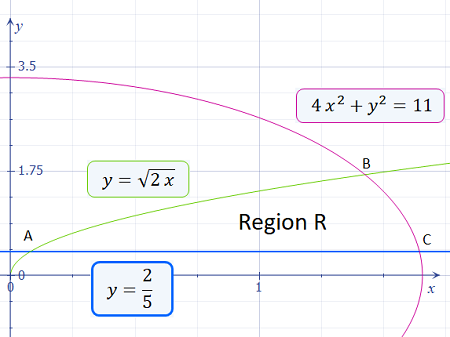# Let R be the region in the first quadrant bounded by the lines y = 2 / 5 and y =\sqrt{2x} and...

## Question:

Let R be the region in the first quadrant bounded by the lines {eq}y = 2 / 5{/eq} and {eq}y =\sqrt{2x}{/eq} and the ellipse {eq}4x^{2}+y^{2}=11{/eq} plot the curves on the same plot to answer questions (a) and (b) below.

a. Use set notation to describe {eq}R{/eq} as a type II region.

(b) Use set notation to describe {eq}R{/eq} as a type I region.

## Type I and Type II Regions:

Let {eq}R{/eq} be a region in the xy-plane. Based on how the region is defined, {eq}R{/eq} can be classified as a Type I or Type II Region such that:

• {eq}R{/eq} is a Type I Region if it can be defined as {eq}R = \left\{\, (x,y) \,|\, a \le x \le b \text{ and } f(x) \le y \le g(x) \,\right\} {/eq}; and,
• {eq}R{/eq} is a Type II Region if it can be defined as {eq}R = \left\{\, (x,y) \,|\, c \le y \le d \text{ and } p(y) \le x \le q(y) \,\right\} {/eq}

## Answer and Explanation:

{eq}R{/eq} is the region in the first quadrant bounded by the lines {eq}y = \frac{2}{5}{/eq} and {eq}y = \sqrt{2x}{/eq} and the ellipse {eq}4x^2 + y^2 = 11{/eq}, as shown in the figure below:Solving for the intersection points A, B and C:

{eq}\begin{align*} &y = \sqrt{2x} = \frac{2}{5} & \text{[Intersection A]} \\ &\Rightarrow 2x = \frac{4}{25} \\ &\Rightarrow x = \frac{2}{25} \\ &\Rightarrow y = \sqrt{2 \left(\frac{2}{25}\right)} \\ &\Rightarrow y = \sqrt{ \frac{4}{25} } \\ &\Rightarrow y = \frac{2}{5} & \boxed{\text{A } \left( \frac{2}{25}, \frac{2}{5} \right) } \\ \\ &4x^2 + y^2 = 11 & \text{[Intersection B]} \\ &\Rightarrow 4x^2 + \left(\sqrt{2x}\right)^2 = 11 & \text{[Subtitute } y = \sqrt{2x} \text{]}\\ &\Rightarrow 4x^2 + 2x - 11 = 0 & \text{[Use the Quadratic Formula]} \\ &\Rightarrow x = \frac{ -2 \pm \sqrt{180}}{8} & \text{[But } x > 0 \text{ since } R \text{ is in QI]} \\ &\Rightarrow x = \frac{ -1 + 3\sqrt{5}}{4} & \text{[Substitute } x \text{ into } y = \sqrt{2x} \text{]} \\ &\Rightarrow y = \sqrt{2 \left(\frac{ -1 + 3\sqrt{5}}{4} \right)} \\ &\Rightarrow y = \frac{\sqrt{-2 + 6\sqrt{5}} }{2} & \boxed{\text{B } \left( \frac{ -1 + 3\sqrt{5}}{4}, \frac{\sqrt{-2 + 6\sqrt{5} } }{2} \right) } \\ \\ &4x^2 + y^2 = 11 & \text{[Intersection C]} \\ &\Rightarrow 4x^2 + \left(\frac{2}{5}\right)^2 = 11 & \left[ \text{Substitute } y = \frac{2}{5} \right] \\ &\Rightarrow 4x^2 = 11 - \frac{4}{25} \\ &\Rightarrow 4x^2 = \frac{271}{25} \\ &\Rightarrow x = \pm \sqrt{\frac{271}{100}} & \text{[But } x > 0 \text{ since } R \text{ is in QI]} \\ &\Rightarrow x = \frac{ \sqrt{271}}{10} & \boxed{\text{C } \left( \frac{ \sqrt{271}}{10}, \frac{2}{5} \right) } \\ \end{align*} {/eq}

A. Type I Region

Rewriting the equation of the ellipse as {eq}y{/eq} in terms of {eq}x{/eq}:

{eq}\begin{align*} &4x^2 + y^2 = 11 \\ &\Rightarrow y^2 = 11 - 4x^2 \\ &\Rightarrow y = \pm \sqrt{ 11 - 4x^2} & \text{[Take the positive root since } R \text{ is in QI] } \\ &\Rightarrow y = \sqrt{ 11 - 4x^2} \end{align*} {/eq}

Therefore, {eq}\boxed{\text{the region can be defined as a Type I Region as } R = \left\{\, (x,y) \,|\, \frac{2}{5} \le y \le \sqrt{2x} \text{ when } \frac{2}{25} \le x \le \frac{ -1 + 3\sqrt{5}}{4} \Cap \frac{2}{5} \le y \le \sqrt{11-4x^2} \text{ when } \frac{ -1 + 3\sqrt{5}}{4} \le x \le \frac{ \sqrt{271}}{10} \,\right\} } {/eq}

B. Type II Region

Rewriting the equation of the curve {eq}y = \sqrt{2x}{/eq} and the ellipse as x in terms of y:

{eq}\begin{align*} &y = \sqrt{2x} \\ &\Rightarrow y^2 = 2x \\ &\Rightarrow x = \frac{y^2}{2} \\ \\ &4x^2 + y^2 = 11 \\ &\Rightarrow 4x^2 = 11 - y^2 \\ &\Rightarrow x = \frac{\sqrt{ 11 - y^2}}{2} \end{align*} {/eq}

Therefore, {eq}\boxed{\text{the region can be defined as a Type II Region as } R = \left\{\, (x,y) \,|\, \frac{2}{5} \le y \le \frac{\sqrt{-2 + 6\sqrt{5} } }{2} \text{ and } \frac{y^2}{2} \le x \le \frac{\sqrt{ 11 - y^2}}{2} \,\right\} } {/eq}

#### Learn more about this topic:Double Integrals & Evaluation by Iterated Integrals

from GRE Math: Study Guide & Test Prep

Chapter 15 / Lesson 4
401